• +91 9971497814
• info@interviewmaterial.com

# Surface Areas and Volumes Ex-13.5 Interview Questions Answers

### Related Subjects

Question 1 :

A copper wire, 3 mmin diameter, is wound about a cylinder whose length is 12 cm, and diameter 10cm, so as to cover the curved surface of the cylinder.

Answer 1 : Find the length and mass of the wire, assuming the density of copper to be 8.88 g per cm_3.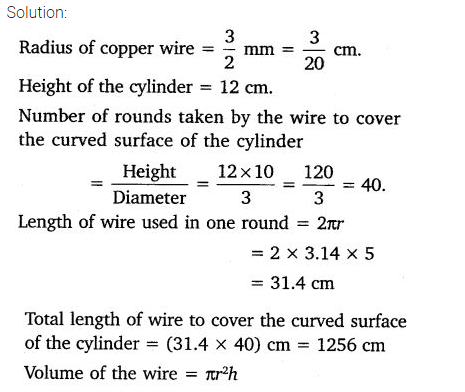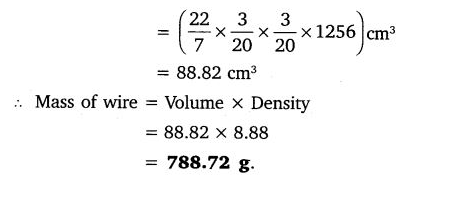Question 2 :

A right trianglewhose sides are 3 cm and 4 cm (other than hypotenuse) is made to revolve aboutits hypotenuse. Find the volume and surface area of the double cone so formed.(Choose value of π as found appropriate)

Answer 2 :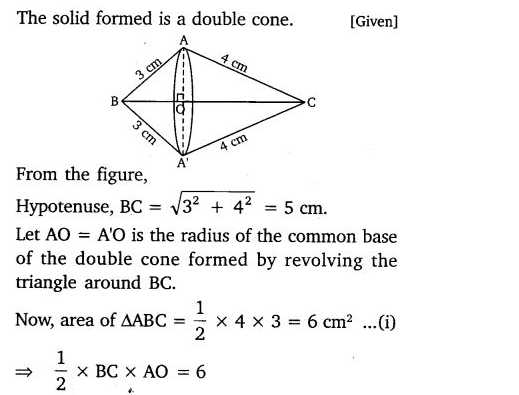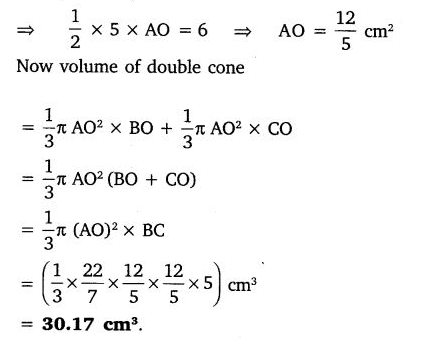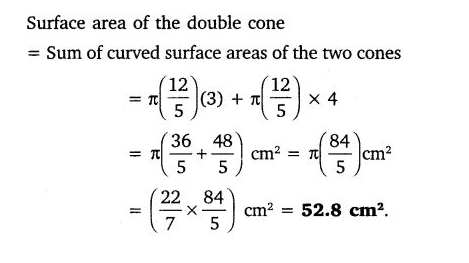Question 3 :

A cistern,internally measuring 150 cm × 120 cm × 100 cm, has 129600 cm3 ofwater in it. Porous bricks are placed in the water

Answer 3 :  until the cistern is full to the brim. Each brick absorbs one-seventeenth of its own volume of water. How many bricks can be put in without overflowing the water, each being 22.5 cm × 7.5 cm × 6.5 cm?

Solution:

Given that the dimension of the cistern = 150× 120 × 110

So, volume = 1980000 cm3

Volume to be filled in cistern = 1980000 –129600

= 1850400 cm3

Now, let the number of bricks placed be “n”

So, volume of n bricks will be =n×22.5×7.5×6.5

Now as each brick absorbs one-seventeenth ofits volume, the volume will be

= n/(17)×(22.5×7.5×6.5)

For the condition given in the question,

The volume of n bricks has to be equal tovolume absorbed by n bricks + Volume to be filled in cistern

Or, n×22.5×7.5×6.5 =1850400+n/(17)×(22.5×7.5×6.5)

Solving this we get,

n = 1792.41

Question 4 :

In one fortnight ofa given month, there was a rainfall of 10 cm in a river valley. If the area ofthe valley is 97280 km2

Answer 4 : show that the total rainfall was approximately equivalent to the addition to the normal water of three rivers each 1072 km long, 75 m wide and 3 m deep.

Solution:

From the question, it is clear that

Total volume of 3 rivers = 3×[(Surface area ofa river)×Depth]

Given,

Surface area of a river = [1072×(75/1000)] km

And,

Depth = (3/1000) km

Now, volume of 3 rivers =3×[1072×(75/1000)]×(3/1000)

= 0.72 km3

Now, volume of rainfall = total surface area ×total height of rain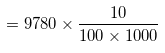= 9.7 km3

For the total rainfall was approximatelyequivalent to the addition to the normal water of three rivers, the volume ofrainfall has to be equal to volume of 3 rivers.

But, 9.7 km3 ≠ 0.72 km3

So, the question statement is false.

Question 5 :

An oil funnel madeof tin sheet consists of a 10 cm long cylindrical portion attached to a frustumof a cone. If the total height is 22 cm,

Answer 5 :  diameter of the cylindrical portion is 8 cm and the diameter of the top of the funnel is 18 cm, find the area of the tin sheet required to make the funnel (see Fig.).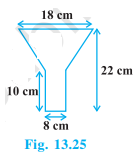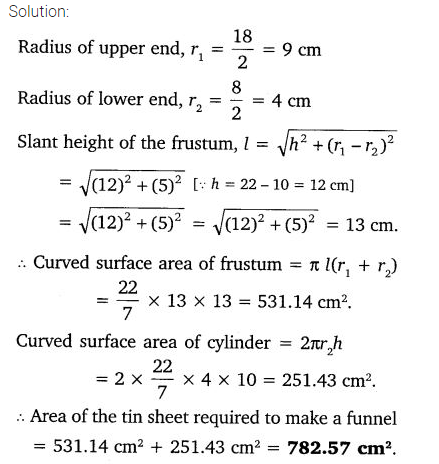Todays Deals### Surface Areas and Volumes Ex-13.5 Contributorskrishan

Name:
Email:

# Latest News# 9000 interview questions in different categories